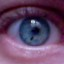# An unpleasant calculation?

At the end of my previous post I claimed that proving that $latex \sum_{s=2}^k \frac{{k \choose s} {n-k \choose k-s}}{{n \choose k}} 2^{{s \choose 2}} ,$ tends to $latex 0$ when $latex n$ tends to infinity and $latex k=(2-\epsilon)\log_2(n)$ was unpleasant. It appears that I was wrong, and I would like to show here a simple proof that was suggested to me by my colleague Philippe Rigollet.

The key observation is that the term $latex \frac{{k \choose s} {n-k \choose k-s}}{{n \choose k}}$ is the probability that an hypergeometric random variable $latex H(k,k,n)$ takes value $latex s$. Hypergeometric distributions are nasty, but when $latex n$ is of larger order than $latex k$ (which is the case here), they are well approximated by a simple binomial $latex B(n, k/n)$. In the present case this suggests the upper bound $latex \frac{{k \choose s} {n-k \choose k-s}}{{n \choose k}} \leq {k \choose s} \left(\frac{k}{n}\right)^s .$ The latter inequality can be proved formally using the identity $latex {n-1 \choose k-1} = \frac{k}{n} {n \choose k} .$ Now one can simply use the inequalities $latex 2^{{s \choose 2}} \leq 2^{s \frac{k}{2}},$ and $latex {k \choose s} \leq k^s$ to obtain that the original unpleasant sum is upper bounded by $latex \sum_{s=2}^{k} 2^{s (\frac{k}{2} + 2\log_2(k) – \log_2(n) )}$ which clearly tends to $latex 0$ when $latex n$ tends to infinity and $latex k=(2-\epsilon)\log_2(n)$.

This entry was posted in Random graphs. Bookmark the permalink.

## One Response to "An unpleasant calculation?"

•